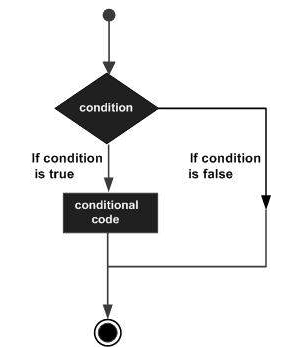# Decision Making in C++

Decision making structures require that the programmer specify one or more conditions to be evaluated or tested by the program, along with a statement or statements to be executed if the condition is determined to be true, and optionally, other statements to be executed if the condition is determined to be false.

Following is the general form of a typical decision-making structure found in most of the programming languages −## If-Else Statement

An if statement can be followed by an optional else statement, which executes when the boolean expression is false. The syntax of an if...else statement in C++ is −

if(boolean_expression) {
// statement(s) will execute if the boolean expression is true
} else {
// statement(s) will execute if the boolean expression is false
}

## Example Code

Live Demo

#include <iostream>
using namespace std;

int main () {
// local variable declaration:
int a = 100;

// check the boolean condition
if( a < 20 ) {
// if condition is true then print the following
cout << "a is less than 20;" << endl;
} else {
// if condition is false then print the following
cout << "a is not less than 20;" << endl;
}
cout << "value of a is : " << a << endl;
return 0;
}

## Output

a is not less than 20;
value of a is : 100

## Switch-Case Statement

A switch statement allows a variable to be tested for equality against a list of values. Each value is called a case, and the variable being switched on is checked for each case. The syntax for a switch statement in C++ is as follows −

switch(expression) {
case constant-expression :
statement(s);
break; //optional
case constant-expression :
statement(s);
break; //optional

// you can have any number of case statements.
default : //Optional
statement(s);
}

## Example Code

Live Demo

#include <iostream>
using namespace std;

int main () {
// local variable declaration:

case 'A' :
cout << "Excellent!" << endl;
break;
case 'B' :
case 'C' :
cout << "Well done" << endl;
break;
case 'D' :
cout << "You passed" << endl;
break;
case 'F' :
cout << "Better try again" << endl;
break;
default :
cout << "Invalid grade" << endl;
}
}
You passed
Your grade is D